TitleCollege Algebra
Answer/Discussion to Practice Problems
Tutorial 54B: SeriesAnswer/Discussion to 1a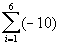What values will you be plugging in to get the terms that will be summed? If you said 1 through 6 you are right on!!!  Let’s see what we get when we add up terms plugging in 1, 2, 3, 4, 5, and 6 for i: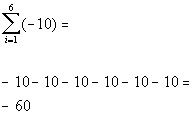Note that the general term is -10, which is a constant.  So no matter what i is, the term is always -10.  Since we were going from 1 to 6, we had 6 terms of -10 be added or 6(-10) = -60.  In general, if your general term is constant than the sum will end up being the number of terms in your series times the constant.Answer/Discussion to 1b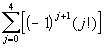What values will you be plugging in to get the terms that will be summed? If you said 0 through 4 you are right on!!!  Let’s see what we get when we add up terms plugging in 0, 1, 2, 3, and 4, for j: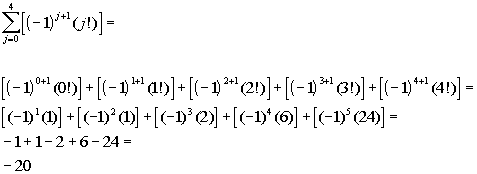If you need a review on factorials, feel free to go to Tutorial 54A: Sequences.Answer/Discussion to 2a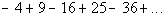There are two things that change.

First let's look at the alternating signs:

 The first term is negative, the second term is positive, the third negative, the fourth positive and so forth. When i is odd (1, 3, 5, ...), then the term is negative. When i is even (2, 4, 6, ...), then the term is positive.  So do you think we are going to have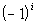or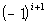. If you saidyou are correct!!!  If n is odd, then this term will be negative.  If n is even, then this term will be positive.

Next, let’s look at the number on each term.

We need to figure out the relationship between i and the exponent:

We need to figure out the relationship between i and the number:

 When i is 1, the number is 4 When i is 2, the number is 9. When i is 3, the number is 16. When i is 4, the number is 25. When i is 5, the number is 36. And so forth....

What do you think the relationship is?
It looks like we have a series of perfect squares.  However, since we have to start with i = 1, we will have to adjust it so that the first term has a 4 in it.

So how will we represent the number?
If you said i + 1, give yourself a pat on the back.

Putting it together,  we can write the summation as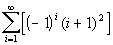Sometimes you have to play around with it before you get it just right.  You can always check it by putting in the i values and seeing if you get the given series.

This one does check.Answer/Discussion to 3aWe need to rewrite the series so that they are both equivalent to each other, but the first one starts at i = 1 and the second one starts at j = 4. We need to find the relationship between n and j and rewrite n in terms of j: Looking at the lower limits for each, how would you write n in terms of j?  What is their relationship? If you said i = j - 3, you are correct!!!  Basically, we need to do a substitution.  Wherever we have an n in our general term, we will replace it with j - 3: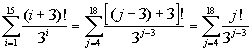Answer/Discussion to 4a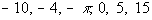Note that I will be rounding to 3.14. We need to find the mean, so we will be adding all of the values that we have and dividing that sum by what number? If you said 6, you are correct! Let’s see what we get: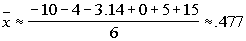Last revised on May 16, 2011 by Kim Seward.
All contents copyright (C) 2002 - 2011, WTAMU and Kim Seward. All rights reserved.